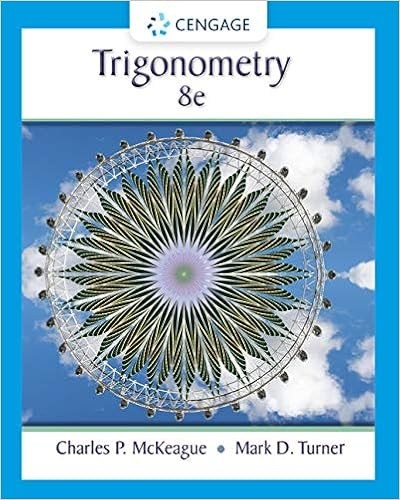Directions: Show all work as shown in class and write the...

• Test Prep
• 4

This preview shows page 1 - 2 out of 4 pages.

We have textbook solutions for you!
The document you are viewing contains questions related to this textbook.The document you are viewing contains questions related to this textbook.
Chapter 7 / Exercise 47
Trigonometry
McKeague/TurnerExpert Verified
Name ____________________ Review for the Final Directions: Show all work as shown in class and write the answers in the spaces provided.
1) Review questions from the homework, quizzes and the practice midterm. 2) Find all real numbers that satisfy the equation 1 cos3 2 s . 3) Find the exact value: 1 arccot 3 4) Find the exact value: 1 cos arcsin 2 5) Find all the angles in 0 ,360 that satisfy the equation 3 tan 1 0 x 6) Find the inverse and state the domain and range of 1 : f   4 tan 2 x f x for 1 1 x   7) Find the exact value(s) for x in the interval , : 2 2
We have textbook solutions for you!
The document you are viewing contains questions related to this textbook.The document you are viewing contains questions related to this textbook.
Chapter 7 / Exercise 47
Trigonometry
McKeague/TurnerExpert Verified
•••